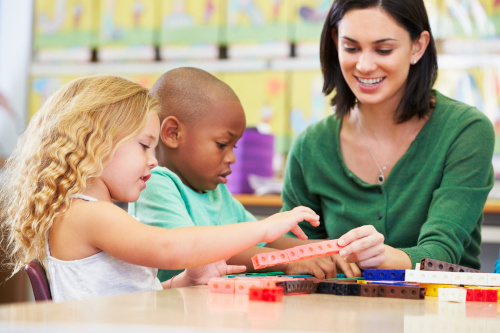# 3 ways to teach multi-sensory math

### Multi-sensory learning enables students to interact with the mathematics for deep, grounded learning

Learning mathematics is much more than memorization. Rote drill and practice have not shown to lead to significant improvements in mathematics abilities, but rather, using strategies that engage and strengthen the connections to different areas of the brain assists students in learning mathematics.

According to findings published in Teaching Children Mathematics, most students actually use a strategy to recall a fact. They are considered fluent if they can recall a fact within three seconds, which is a long time to be able to employ a strategy. For example, looking at the problem 19 + 6, students might move one from the 6 to make an easier, equivalent statement of 20 + 5.

Using multi-sensory learning to make sense of mathematics, as well as introducing students to strategies and tools such as the ones below, helps them become flexible thinkers and allows them to be fluid with numbers.

Counters and ten frames

Counters and ten frames help students “see” ten. Students use the counters and fill spaces in a ten frame. When they have all ten spaces full, they can see that they have ten. It is very satisfying!

If there are two empty spaces, they can see they have less than ten. Teachers can ask a question like, “How many more do you need to make ten?” Students can count on and realize they need two. Later, teachers can connect this visual model to the much more abstract number model of 8 + 2 = 10, and even 10 = 8 + 2.

Want to share a great resource? Let us know at submissions@eschoolmedia.com.